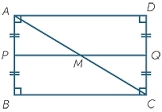# Rectangular 9241

Calculate the area of ​​a rectangular garden if it consumed 266 ordinary meters of mesh on its fencing and if we know that the difference between the lengths of two adjacent sides of the garden is 15 m.

S =  4366 m2

### Step-by-step explanation:Did you find an error or inaccuracy? Feel free to write us. Thank you!

Tips for related online calculators
Do you have a linear equation or system of equations and looking for its solution? Or do you have a quadratic equation?
Do you want to convert length units?

#### Grade of the word problem:

We encourage you to watch this tutorial video on this math problem: